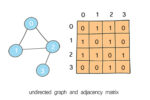A graph is a data structure that consists of a set of  nodes connected by edges. Graphs are used to simulate many real-world problems, such as paths in cities, circuit networks, and social networks. This is graph implementation part 3 – graph as adjacency matrix.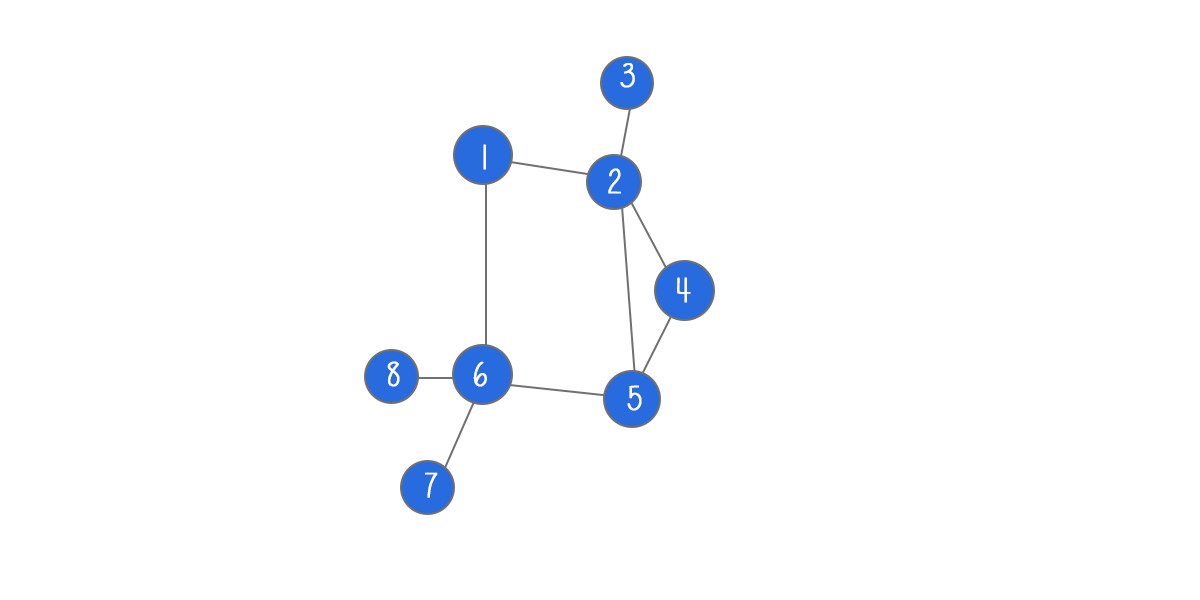### Terminology

A node in the graph is also called vertex.  A line between two nodes is edge. Two nodes are adjacent (or neighbors) if they are connected to each other through an edge. Path represents a sequence of edges between the two nodes.

In a directed graph, all of the edges represent a one-way relationship. In an undirected graph, all edges are bi-directional.

If the edges in the graph have weights, the graph is said to be a weighted graph. If the edges do not have weights, the graph is said to be unweighted.

Graph can be presented as adjacency list or adjacency matrix. An adjacency list is an array of edges or nodes. Adjacency list is used for representation of the sparse graphs. An adjacency matrix is a square matrix with dimensions equivalent to the number of nodes in the graph. Adjacency matrix is preferred when the graph is dense.

An adjacency matrix is a 2D array in which the elements indicate whether an edge is present between two nodes. If a graph has N nodes, the adjacency matrix is NxN array.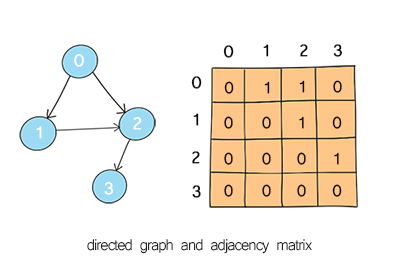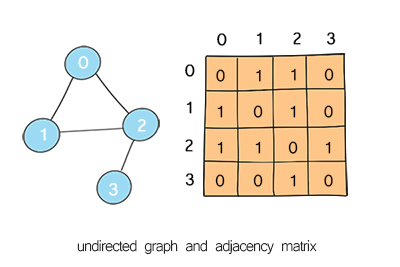### Map of graph implementationsPart 3 – Graph as adjacency matrix

### Define class and initialize

This is to initialize the graph from an input matrix. The nodes are the indices for both rows and columns. An edge between two nodes is indicated at the cell [r][c]. The value can be a number, character or object. If it is a number and the number is not 0, it indicates the weight of the edge.

If a graph is directed, the value will be assigned at cell [r][c]. If a graph is undirected, the value will be assigned at cell[c][r] as well.

If two nodes have no connections, the cell will be marked with different values. Note in this implementation, the input parameter blockValue is to specify this value, meaning the cell is blocked or cannot pass through.

After initialization, the number of rows and number of columns are defined. The value of elements in the matrix are assigned.

## Python

### Depth first search in graph as adjacency matrix

The same as graph as adjacency list, find path from source to destination in matrix can be done using depth first search (DFS) and breadth first search (BFS).

Depth First Search starts from the source node, and explores all adjacent cells with recursion. The adjacent cells can be accessed by increasing or decreasing the index of the row and column. For example if the current position is [r][c], the adjacent cells are [r-1][c] , [r+1][c], [r][c-1], [r][c+1], which means up, down, left and right.

To avoid visiting the same cell repeatedly, a 2D boolean variable visited is used. It is to track whether the cell has been visited. If a cell has been visited, it should not be visited again. This will keep the time complexity down to O(m*n). This technique is called memoization.

## Doodle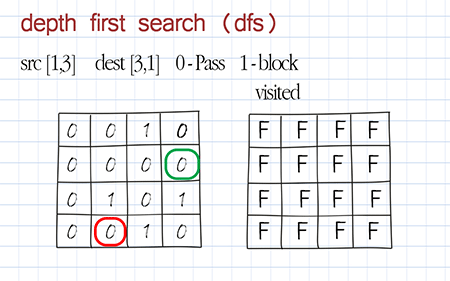### Breadth first search in graph as adjacency matrix

Breadth First Search starts from the source node, and explores all its adjacent nodes before going to the next level adjacent nodes. A queue is used to keep track the sequence to visit.

The same as DFS, 4 adjacent cells are accessed by increasing and decreasing index, ie [r-1][c] , [r+1][c], [r][c-1], [r][c+1]. The 2D boolean variable visited are functioning the same way as DFS.

## Doodle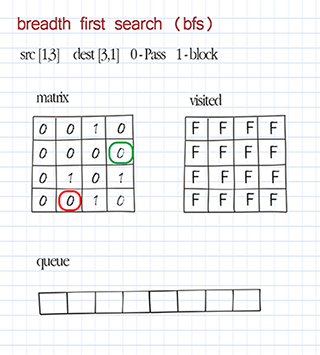### Print graph as adjacency matrix

Print is to print all values in the graph as adjacency matrix. This method is used for debugging purpose.

## Python